## The Double Slit Experiment

Let's start with a concrete experiment as the basis of the discussion following: let's take a look at the main features of the so-called double slit experiment.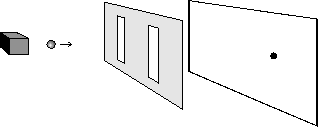Figure 1: A single particle, both slits are open.

If we let single particles from an appropriate source hit a screen with two parallel slits, then we observe single black spots on the photo plate behind the screen.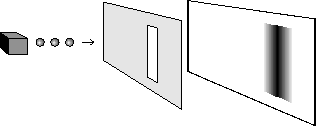Figure 2: Many particles, one slit is open.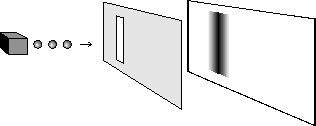Figure 3: Many particles, one slit is open.

If we close one of the slits and repeat the experiment many times (equivalently, we might use a beam of particles), then we find a black strip on the photo plate which we may understand as an image of the open slit.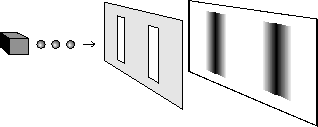Figure 4: Many classical particles, both slits are open.

If the particles obeyed the classical, i.e. Newtonian, laws of motion, then on the photo plate simply two strips should appear as soon as both slits are open.

But if we carry out this experiment with particles from the atomic realm, we find an interference pattern on the photo plate, similar to those observed at interfering light waves.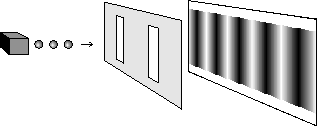Figure 5: Many atomic particles, both slits are open.

So the motion of particles in the atomic realm does not obey the classical laws of motion.

Thus, in the realm of atomic particle we have the following situation: Single particles pass the double slit one by one and produce single spots on the photo plate. In total, however, the spots lead to interference patterns, usually known only in the context of waves. Therefore one speaks of a wave-particle dualism.

#### The Double-Slit Experiment in Quantum Mechanics

In quantum mechanics, atomic objects like electrons have properties of both waves and particles. The usual understanding of wave-particle dualism is that an electron must be described as a wave while it passes through the two slits; only when we force it with the photo plate to disclose its position, it behaves like a particle and causes a black spot.

Thus quantum mechanics is not a theory describing the motion of point-shaped particles. On the contrary, QM makes statements on the probabilities of results of experiments. For the double-slit experiment, e.g., it correctly describes the shape of the interference pattern, but not the way on which a single particle arrives at the black spot, and not how the spot came about.

The notion of the path of a particle does not have any meaning in quantum mechanics.

Werner Heisenberg

The following quotation draws attention to the deep-rooted problems of quantum mechanics which already show up in the explanation of the double-slit experiment:

We choose to examine a phenomenon which is impossible, absolutely impossible, to explain in any classical way, and which has in it the heart of quantum mechanics. In reality it contains the only mystery. We cannot make the mystery go away by `explaining' how it works.

Richard P. Feynman, on the double-slit experiment

## Bohmian Mechanics

Bohmian mechanics -- named after physicist David Bohm, who founded this theory in 1952 -- is a deterministic, Galilei-invariant (i.e. nonrelativistic) theory of the motion of point particles.

Bohmian mechanics and nonrelativistic quantum mechanics make the same predictions concerning the results of experiments.

Quantum mechanics plays the role of a ``phenomenological'' measurement formalism of Bohmian mechanics; by this the problems and paradoxies of quantum mechanics can be solved.

And here is, in outline, how the dynamics of Bohmian particles work. The particles move along paths which are determined by a wave. Similar to surf riding on a water wave, they are guided by Schrödingers wave function. Below you find the mathematical formulation of this picture.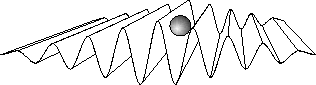Figure 6: An (actually point-shaped) particle is guided by a wave on some high dimensional space, the configuration space.

#### The Double-Slit Experiment in Bohmian Mechanics

As Newtonian mechanics, Bohmian mechanics describes the motion of particles along paths. In this sense, it is a ``classical'' theory, but of course with different equations of motion. Nevertheless, it is able to reproduce correctly the observations of the double-slit experiment.

In contrast to quantum mechanics, BM does not only provide the shape of the interference pattern, but also information about the paths of single particles. In Fig. 7, some possible paths of Bohmian particles behind the double slit are drawn.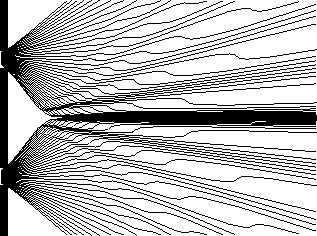Figure 7: Bohmian paths in the double-slit experiment.

One sees immediately that the paths are non-Newtonian, i.e. the particles do not move on straight lines in the region behind the slits, but gather at the maxima of the Schrödinger wave.

Indeed the shape of the wave function is the same for all particles, but as they start from different positions, they arrive at different positions on the plate.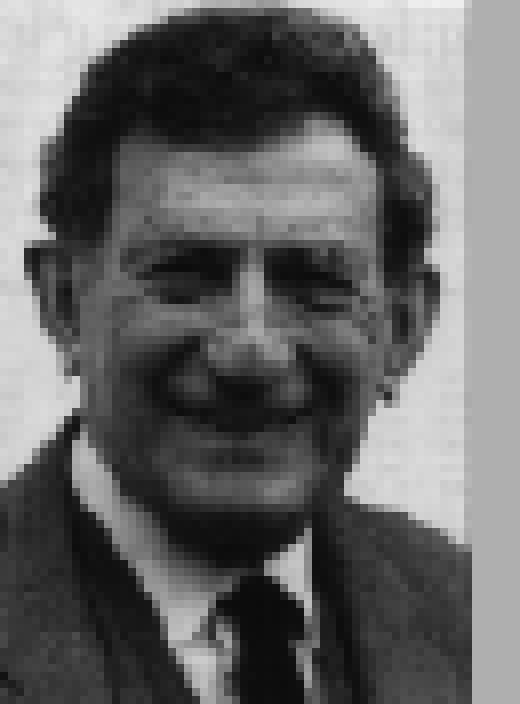Figure 8: David Bohm (1917-1992) in 1989. Eventually, he taught at Birkbeck College in London.

#### The Theory: Bohmian Mechanics

The state of a physical system of N particles with masses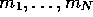is represented in Bohmian mechanics by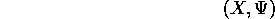where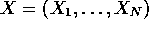are the spatial coordinates of the N particles and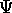is Schrödinger's wave function.

The temporal change of the wave function is governed by the Schrödinger equation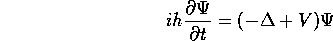.

In Bohmian mechanics, the job of the wave function is to ``guide'' the particles, i.e. the wave function generates a velocity field which the particles follow. Mathematically, this is expressed by a first-order differential equation: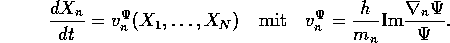Actually, this is the most simple possibility for a Galilei-invariant theory of motion of particles. The theory directly specifies the rate of change of particle positions.

In contrast, in Newtonian mechanics the rate of change of the velocities is specified, that is the change of the change of the positions. Instead of a velocity field, there we have an ``acceleration field'', usually called a force field. Newton's law reads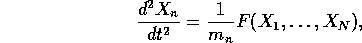where F denotes the force.

## Research Topics

Bohmian mechanics explains quantum mechanics, just like statistical mechanics is the foundation of thermodynamics. The mathematically and physically precise examination of this connection is one of the research topics of our group. Here is what sort of questions have been treated and what sort of statements have been proved:

• For a large class of Bohmian systems, the dynamics exist for all times. The analogue statement about Newtonian mechanics could not be established up to now.
• The operator formalism of quantum mechanics arises as a consequence from Bohmian mechanics as a ``measurement formalism'': a big Bohmian system behaves like quantum mechanics predicts.
• As well, the quantum mechanical scattering theory, the classical limit and the question about time measurements in quantum mechanics have been investigated on by our group.

The second field of research of our group are relativistic particle theories:

• Bohmian mechanics, just like quantum mechanics, provides the correct physics only in the nonrelativistic realm, that means for small velocities as compared to the speed of light. That's why we try to construe a relativistic particle theory whose nonrelativistic limit is Bohmian mechanics.
• For a certain existing relativistic particle theory, so-called Feynman-Wheeler electrodynamics, the global existence of solutions has been established.

## Quantum-Mechanical Measurement of Time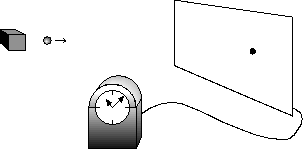Figure 9: The same experiment with a mild modification: we removed the double slit and connected the plate to a clock that displays when the electron arrived.

Let's remove the slits and ask: When does the electron arrive at the plate?

If it was for billard balls, the answer would be immediate:

time = distance/velocity, t = s/v.

For electrons, this formula cannot be applied because of the uncertainty relation.

Time measurement is an example for the difficulties of quantum mechanics: the above experiment can certainly be carried out, but standard methods of quantum mechanics cannot compute the result (``there is no time operator'').

In Bohmian mechanics, we may calculate when the electron arrives at the detector (if we know precisely where it started we can compute the path).

This method, however, is very expendable: we must calculate lots of paths as we can never know where the electron started.

That's why we reformulate the task and ask for the probability that an electron hits the plate during the time interval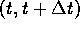.

The situation is comparable to that of a gas: for a gas particle of velocity v to hit a square of edge length a within the intervalit must dwell in a cuboid of size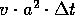. Now multiply this with the density (the number of gas particles per unit volume) -- the product densityvelocity is usually called current and denoted j.

For the electron, too, there exists a current (j is the ``quantum mechanical probability current''), so now we have the probability that the electron arrives on the screen at time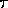: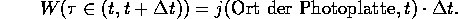This formula is based on the concept of a path and thus cannot be derived within standard quantum mechanics. In our group we study its consequences in Bohmian mechanics.

## Return to Classical Behaviour in Quantum Mechanics

All physical experiments so far indicate that nature obeys the laws of quantum mechanics. Why then don't the bizarre wave effects we learned about at the double-slit experiment appear in everyday life? We get a hint when taking another look at the experiment.

#### The Double Slit Experiment in Vacuum

To find the wave effect, the entire experimental arrangement must be screened very carefully against all external influences (such as air and light). In this case we need to describe only the single electron flying through the set-up, which we do mathematically by determining a function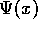that specifies for every position x the amplitude of the wave.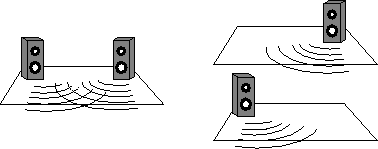Figure 10: Left: Analogously to the double slit in vacuum, the superposition of the pressure waves effectuates the stereo sound. Right: the loudspeakers are far apart in height, so we twice get mono sound. This case corresponds to the double slit with air.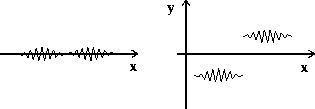Figure 11: Left: The electron in vacuum. The partial waves are close together and may superpose. Right: In air. Because of collisions with gas molecules, the partial waves get separated in configuration space (indicated by the y axis). There is no superposition any more.

Now this wave meets the wall with the two slits, and each slit lets pass a part of the wave. Behind the wall, the two partial waves meet again and superpose, just like, e.g., sound waves from two loudspeakers do. In the case of the loudspeakers, the notes come about by the superposition of the waves from both loudspeakers. Analogously, the motion of the particle in the experiment is influenced by the superposition of the two partial waves, such that on the screen the typical wave pattern appears.

#### The Double Slit Experiment in Air

Now let there be, beyond the electron, air between the doubly slitted wall and the absorbing screen. Every gas molecule is described by a wave as well. The amplitude of the total wave depends on the entire configuration, i.e. not only on the position x of the electron, but also on the positions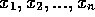of all gas molecules. As a consequence, the wave is not a function of 3-dimensional space (corresponding to the position of the electron), but of a higher-dimensional, so-called configuration space (corresponding to the positions of the electron and all the gas molecules).

As before, through each of the two slits a part of the electron wave passes. Each partial wave collides, on its way, with many gas molecules and thus changes their positions. It is crucial that (as seen in Fig. 12) the two partial waves change the molecule positions in a different way.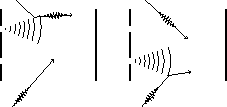Figure 12: The partial wave passing through the upper slit influences the wandering gas molecules in a way different from the partial wave passing through the lower slit.

Hence the partial waves correspond to far apart locations in configuration space. They cannot superpose any more. In the context of loudspeakers, this means that one of the loudspeakers is located on a different floor. The emitted sound waves are equal, but the stereo effect is gone. You can only hear either one or the other. Similarly, the electron particles of our experiment are guided only by one of the two partial waves. The superposition pattern on the screen vanishes, and instead we end up with two stripes as one would have expected classically (Fig. 4).

Classical behaviour of a system comes about only through the interaction with its environment.

## Nonlocality

The Bohmian particles are guided by a wave on configuration space. We have seen that this high-dimensional space, as opposed to the phase space of classical mechanics, is not merely an abstract mathematical tool but an element of the theory crucial to the understanding of the behaviour of Bohmian particles.

In particular, the motion of a particle depends on the positions of all the other particles, no matter how distant they are!

At first sight, this non-local behaviour seems to be in conflict with the principles of the theory of relativity, since we always assumed that physical effects can at most travel at the speed of light.

The seminal work of J.S. Bell however, together with the incompleteness argument of Einstein, Podolsky and Rosen, shows that every theory that agrees with the statistics predicted by quantum mechanics (and thus with experiment) must be nonlocal.

#### The EPR Argument

Einstein, Podolsky and Rosen showed with a thought experiment that quantum theory is incomplete. Bohm developed a simplified version which serves as a basis for actual experiments.

We prepare a system of two spin-particles in the so-called singlet state which fly apart in opposite directions. In two widely separated Stern-Gerlach magnets, the particles ar deflected either to the North or the South pole of the magnet (Fig. 13). Depending on the deflection, one says the particle ``has spin up'' or ``has spin down''.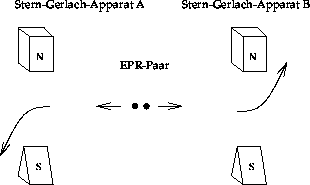Figure 13: Given equal orientations of the magnets, the particles of an EPR pair are deflected in opposite directions.

One observes that whenever the spatial orientation of the two magnets coincides, the two particles are deflected in opposite directions. And the orientations of the magnets can be chosen at late times, such that no effect travelling at the speed of light can allow the particles to ``communicate''. Assumed locality, it is impossible for a particle to decide about North or South only when passing the magnet, as it could not manage to inform in time the other particle which must choose precisely the opposite direction. Thus the particles must carry the information about which way to go (given an arbitrary orientation of the magnet) all the time, before they reach the magnets. Since quantum mechanics cannot describe this piece of information, it must be incomplete, that is, there must be additional ``hidden parameters'':

EPR:
 Quantum mechanics + locality => There must be local hidden parameters.

#### Bell's Theorem

Bell considers the same experiment but focuses on correlations between results of spin measurement at different orientation of the two magnets.

He then shows that the correlations predicted by quantum mechanics cannot be generated by local hidden parameters:

Bell:
 Quantum mechanics => There can't be local hidden parameters.

Together with the EPR argument, this entails that either quantum mechanics makes wrong predictions or locality does not hold. Since (most of) the experiments confirm the quantum prediction, we conclude:

 Bell + EPR + experiment => Nature is nonlocal. There is instantaneous action-at-a-distance.

Oct 15, 1997. Written by students of the Bohmian mechanics group.